# High School Math : Understanding Indirect Proportionality

## Example Questions

### Example Question #51 : Basic Single Variable Algebra

Ifis inversely proportional to, and ifwhen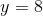, find a formula for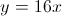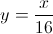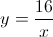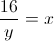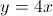Since y is inversely porportional to x, you can use the standard equations of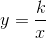where k is a constant. Plugging the given x and y into this equation to solve for k,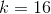. To get the answer, substitute k into the standard equation.